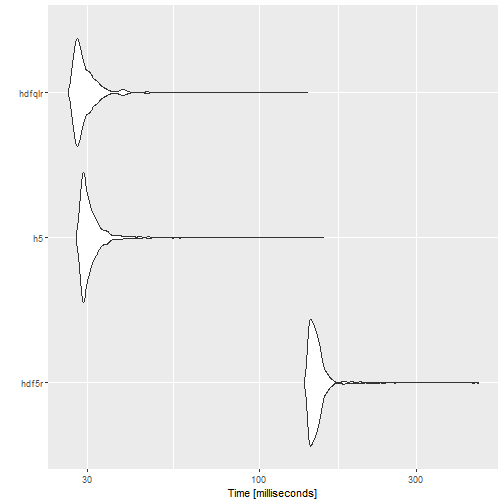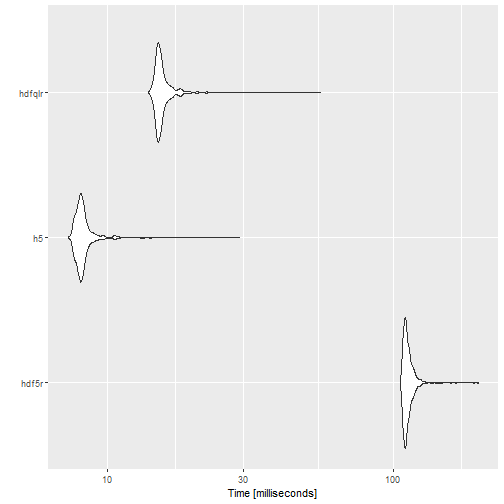# Benchmarking hdfqlr

#### 2019-07-11

This document provides some benchmarks for comparing the performance of hdfqlr to two other HDF file APIs available in R: The hdf5r package and the now-deprecated h5 package. Other packages that provide read (but not write) support for HDF files were not tested.

library(hdfqlr)
library(hdf5r)
library(h5)
library(microbenchmark)

## Writing HDF datasets

write_hdfqlr = function() {
test.file = tempfile(fileext = ".h5")
hql_create_file(test.file)
hql_use_file(test.file)
for (i in paste0("vector", 1:6)) {
write("DATASET", runif(10000), i)
}
hql_close_file(test.file)
}

write_hdf5r = function() {
test.file = tempfile(fileext = ".h5")
# create hdf5 file (6 vectors with 10k random numbers each)
h5file = hdf5r::H5File$new(test.file, "w") for (i in paste0("vector", 1:6)) { h5file[[i]] = runif(10000) } h5file$close_all()
}

write_h5 = function() {
test.file = tempfile(fileext = ".h5")
f = h5::h5file(test.file)
for (i in paste0("vector", 1:6)) {
f[i] = runif(10000)
}
h5::h5close(f)
}

write.benchmark = microbenchmark(
"hdf5r" = write_hdf5r(),
"h5" = write_h5(),
"hdfqlr" = write_hdfqlr(),
times = 1000L,
unit = 'ms'
)
expr min lq mean median uq max neval
hdf5r 137.01529 142.83631 149.75298 146.79678 151.55699 460.1412 1000
h5 27.72461 29.08571 30.89929 29.85698 31.29489 156.5213 1000
hdfqlr 26.27676 27.78007 29.81201 28.59322 30.40774 139.9679 1000plot of chunk write-benchmark

tf = tempfile(fileext = ".h5")
hql_create_file(tf)
hql_use_file(tf)
sets = paste0("vector", 1:6)
for (i in sets) {
hql_write_dataset(runif(10000), i)
}
hql_close_file(tf)

hql_use_file(file)
hql_close_file(file)
result
}

h5file = hdf5r::H5File$new(file, "r") result = lapply(sets, function(i) h5file[[i]][]) h5file$close_all()
result
}

h5file = h5::h5file(file, "r")
result = lapply(sets, function(i) h5file[i][])
h5::h5close(h5file)
result
}

)